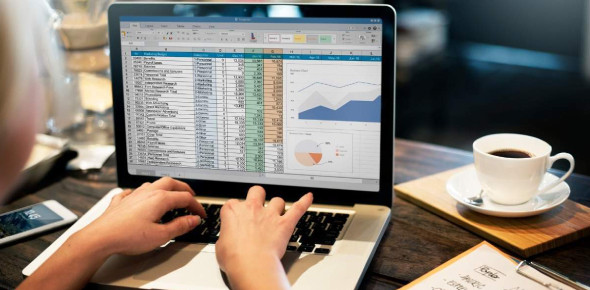# Only An Expert Can Pass This MS Excel Trivia Quiz!

20 Questions | Attempts: 213
ShareSettingsAre you an expert at MS Excel? Only a proficient person can pass this MS Excel Trivia Quiz. You will need to understand what a collected block of data is organized in rows and includes data about a single item. Each column contains one category of data about that item and which function counts items within range and matches a specific condition. This quiz will help put your ducks in a row with MS Excel.

• 1.
A __________ is a collected block of data organized so that each row includes all of the data about a single item and each column contains one category of data about that item.
• A.

Worksheet

• B.

Graph

• C.

Table

• D.

Record

• 2.
A row in a table is also known as a __________ and includes all of the data about a single item.
• A.

Field

• B.

Record

• C.

Category

• D.

Table

• 3.
A column or __________ contains one category of data about a single item.
• A.

Field

• B.

Record

• C.

Category

• D.

Table

• 4.
Excel creates __________ headers to sort and filter data.
• A.

Form

• B.

Cell

• C.

Data

• D.

Table

• 5.
The programming language used to write computer programs within the Microsoft environment is called ________.
• A.

COBOL

• B.

Visual Basic Application

• C.

Basic

• D.

C+

• 6.
Conditions that limit the records displayed are called _________ criteria.
• A.

Condition

• B.

Filter

• C.

Record

• D.

Data

• 7.
Datatase functions are identified by the letter __________.
• A.

A

• B.

B

• C.

C

• D.

D

• 8.
The __________ function determines the average value of a column or field in an Excel table, based on criteria that are set to limit the data.
• A.

DAVERAGE

• B.

DCOUNT

• C.

DSUM

• D.

DIF

• 9.
The __________ function determines the sum of a column of values in an Excel table, based on criteria that are set to limit the data.
• A.

DCOUNT

• B.

DAVERAGE

• C.

DSUM

• D.

DIF

• 10.
A(n) __________ is a of commands that can be recorded and played back later.
• A.

Function

• B.

Argument

• C.

Macro

• D.

Formula

• 11.
The __________ tab is used to work with macros and developers who customize Excel.
• A.

Insert

• B.

Page Layout

• C.

View

• D.

Developer

• 12.
The __________ tab is used to work with macros and developers who customize Excel.
• A.

Security

• B.

Identity

• C.

Theft

• D.

Detection

• 13.
Excel uses a macro __________ to create a macro.
• A.

Function

• B.

Recorder

• C.

Library

• D.

Formula

• 14.
Excel uses a macro __________ to create a macro.
• A.

Rename

• B.

Delete

• C.

Duplicate

• D.

Mark

• 15.
The value of creating a macro is the ability to _________ the macro.
• A.

Copy

• B.

Run

• C.

Edit

• D.

Cut

• 16.
By default, a __________ bar displays security alerts when there is potentially unsafe content in the worksheet that you open.
• A.

Tool

• B.

Message

• C.

Status

• D.

• 17.
A PivotTable report uses the columns of your worksheet to ___________ multiple rows of data.
• A.

Run

• B.

Average

• C.

Summarize

• D.

Display

• 18.
The data in a PivotTable can be updated by using the __________ command.
• A.

Create

• B.

Refresh

• C.

Fields

• D.

Data

• 19.
The ___________ function counts items within a range that match a specific condition.
• A.

COUNTIF

• B.

SUMIF

• C.

AVERAGEIF

• D.

COUNTA

• 20.
The _____ recorder works just like an audio recorder. Every keystroke and mouse click is recorded and saved for future use.
• A.

Security

• B.

Message

• C.

Pivot

• D.

Macro

## Related TopicsBack to top
×

Wait!
Here's an interesting quiz for you.